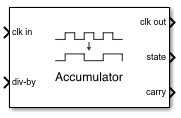Main Content

# Fractional Clock Divider with Accumulator

Clock divider that divides frequency of input signal by fractional number

• Library:
• Mixed-Signal Blockset / PLL / Building Blocks

•## Description

The Fractional Clock Divider with Accumulator block divides the frequency of the input signal by a tunable fractional value (N.FF). When compared to the Single Modulus Prescaler block, the Fractional Clock Divider with Accumulator block helps to achieve a narrow channel spacing that can be less than the reference frequency of a phase-locked loop (PLL) system.

## Ports

### Input

expand all

Input clock frequency, specified as a scalar. In a PLL system, the clk in port is connected to the output port of a VCO block.

Data Types: `double`

Ratio of output to input clock frequency, specified as a fractional scalar.

The value at the div-by port is split into two parts: the integer part (N) and the fractional part (.FF).

Data Types: `double`

### Output

expand all

Output clock frequency, specified as a scalar. In a PLL system, the clk out port is connected to the feedback input port of a PFD block. The output at the clk out port is a square pulse train of 1 V amplitude.

Data Types: `double`

The fractional missing pulse storage. The value of the state port goes up by F with each rising edge of the clk out value of the previous cycle. Whenever the state port value goes over `1`, the value overflows and sets the carry port value to `1`.

Data Types: `double`

Output port that activates the pulse swallow function when state port overflows. The pulse removal is analogous to dividing the input frequency by N+1 instead of N.

Data Types: `Boolean`

## Parameters

expand all

Select to enable increased buffer size during simulation. This increases the buffer size of the Logic Decision inside the Fractional Clock Divider with Accumulator block. By default, this option is deselected.

Number of samples of the input buffering available during simulation, specified as a positive integer scalar. This sets the buffer size of the Logic Decision inside the Fractional Clock Divider with Accumulator block.

Selecting different simulation solver or sampling strategies can change the number of input samples needed to produce an accurate output sample. Set the Buffer size to a large enough value so that the input buffer contains all the input samples required.

#### Dependencies

This parameter is only available when Enable increased buffer size option is selected in the Block Parameters dialog box.

#### Programmatic Use

• Use `get_param(gcb,'NBuffer')` to view the current value of Buffer size.

• Use `set_param(gcb,'NBuffer',value)` to set Buffer size to a specific value.

## More About

expand all

 Best, Roland E. Phase-Locked Loop. New York, NY: Tata McGraw-Hill Companies Inc., 2003.

## SupportGet trial now